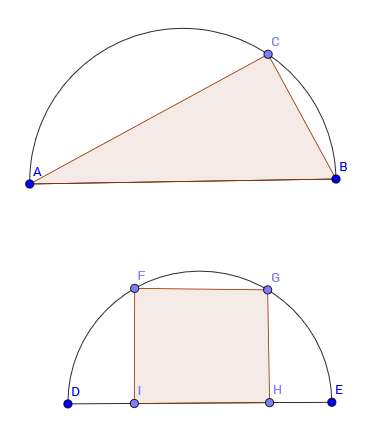# Bonne Chance!

Calculus Level 2A triangle and a rectangle are in semicircles of radius $r$ where two of their vertices are on the diameter, and the rests are on the arc. The angle of vertex $A$ of the triangle is equally probable to be any angle between $0$ and $\dfrac { \pi }{ 2 }$, while for a rectangle the height $GH$ is equally probable to be any height between $0$ and $r$. If you throw a dart and hit a polygon in the semicircle you will win a prize, but you are blindfolded, and you just have one chance. Which polygon will you choose? Why?

Assume that the dart has an equal chance of landing anywhere on the semicircle.

×

Problem Loading...

Note Loading...

Set Loading...